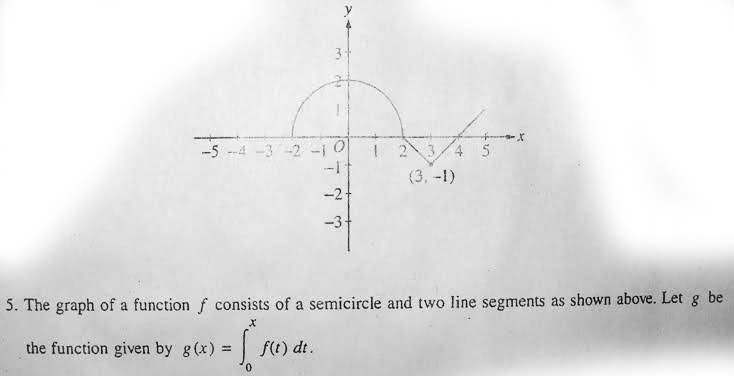# Graph of integral

## Homework StatementFind the x-coordinate of each point of inflection of the graph of g on the open interval (-2, 5). Justify your answer

## The Attempt at a Solution

The answer (I think) is x=0 and x=3. Well, the thing is that I asked my tutor how to justify it and he said that it is only x=0 and not 3. But when I asked my teacher for the answer he checked and said it x=0, 3, but he couldn't tell me how to justify it. So now I'm wondering if I'm right or my tutor is.

The reason why I thought it was x=0 and x=3 is because from -2, the area as you move towards 0 is increasing at a greater and greater rate, therefore it's concave up. Then after 0 it's still increasing, but its rate of increase is decreasing, therefore concave down. Then at 2 it starts decreasing but the magnitude of the rate of decrease is still increasing (therefor still concave down) until x=3. Then after 3 it's still decreasing, but the magnitude of the rate is decreasing so it's concave up again. Then at 4 it starts to increase with increasing rate, and so g is still concave up.

But I'm pretty sure that on an exam they want a quick explanation in mathematical terms, not a whole long paragraph like that. My tutor said that because at x=3 there's a cusp so that's not a solution but I don't get why.## The Attempt at a Solution

Your answers are correct - the key lies in understanding two things:

When do inflection points occur in a function?

How are the functions g and f related?

The answer to the first question (it has something to do with derivatives...) will help you answer the second one (along with the 2nd Fundamental Theorem).

Sorry to be so vague, but I want you to figure it out for yourself.

Inflection points should occur where g''(x)=0...
And g'(x)=f(x), so g''(x)=f'(x), so they should occur where the slope changes from positive to negative or vice versa?

What's your justification that g'(x) is differentiable? Remember, inflection points can happen where g''(x) doesn't exist.